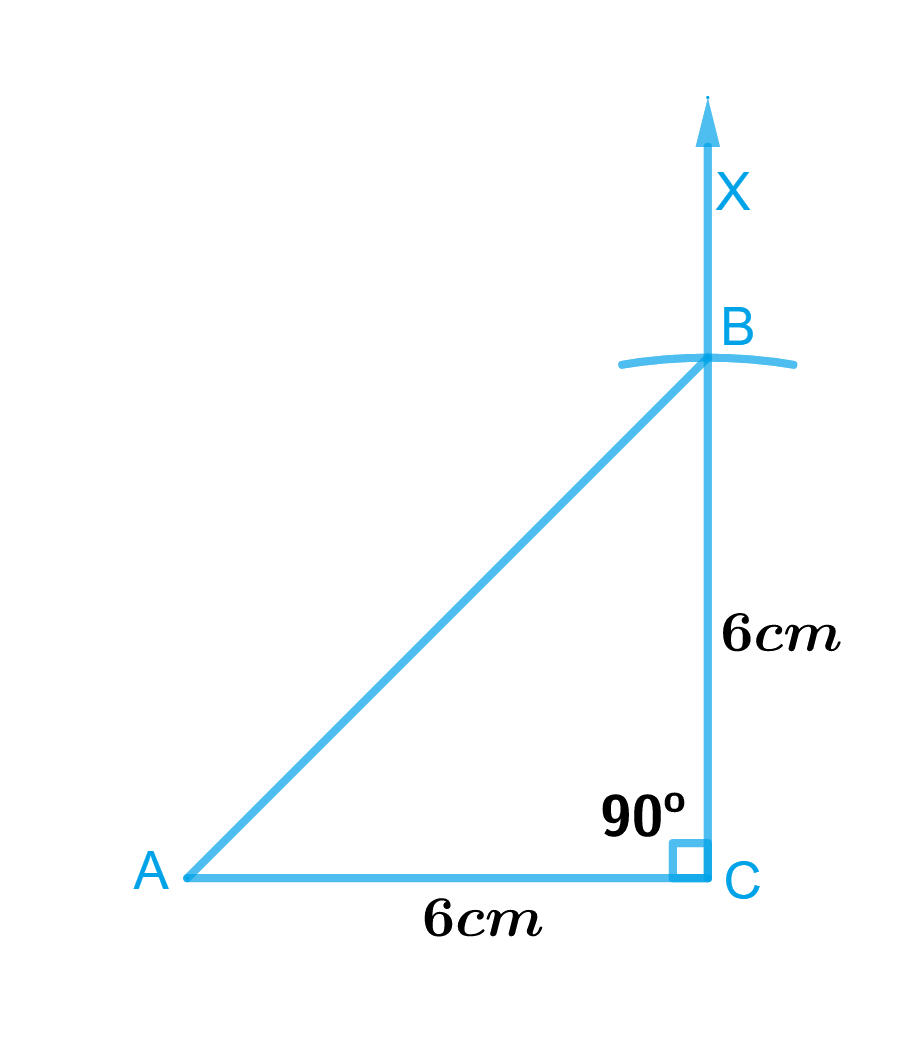# Ex.10.5 Q3 Practical Geometry Solution- NCERT Maths Class 7

## Question

Construct an isosceles right-angled triangle $$ABC$$, where $$m∠ACB = 90^\circ$$ and $$AC = 6\,\rm{ cm}.$$

Video Solution
Practical Geometry
Ex 10.5 | Question 3

## Text Solution

To construct:

An isosceles right-angled triangle $$ABC$$, where $$m∠ACB = 90^\circ$$ and $$AC = 6\,\rm{ cm}.$$

What is known?

Length of side of an isosceles right-angled triangle $$ABC$$,$$AC = 6\,\rm{cm}$$ and $$∠ACB = 90^\circ$$.

Reasoning:

Since $$ΔABC$$ is an isosceles right-angled triangle, where $$∠ACB = 90^\circ$$ and $$AC = 6\,\rm{ cm}$$. Therefore, length of the other equal side, we can take is $$BC = 6\,\rm{cm}$$. To construct this triangle, follow the steps given below.

Steps:Steps of construction

1. Draw a line segment $$CA$$ of length $$6\,\rm{cm}.$$
2. At point $$C,$$ draw $$CX$$ perpendicular $$CA.$$
3. With $$C$$ as centre, draw an arc of radius $$6\,\rm{cm}$$ which should intersect $$CX$$ at point $$B.$$
4. Join $$A$$ and $$B.$$

Triangle $$ABC$$ is the required isosceles right-angles triangle.

Learn from the best math teachers and top your exams

• Live one on one classroom and doubt clearing
• Practice worksheets in and after class for conceptual clarity
• Personalized curriculum to keep up with school